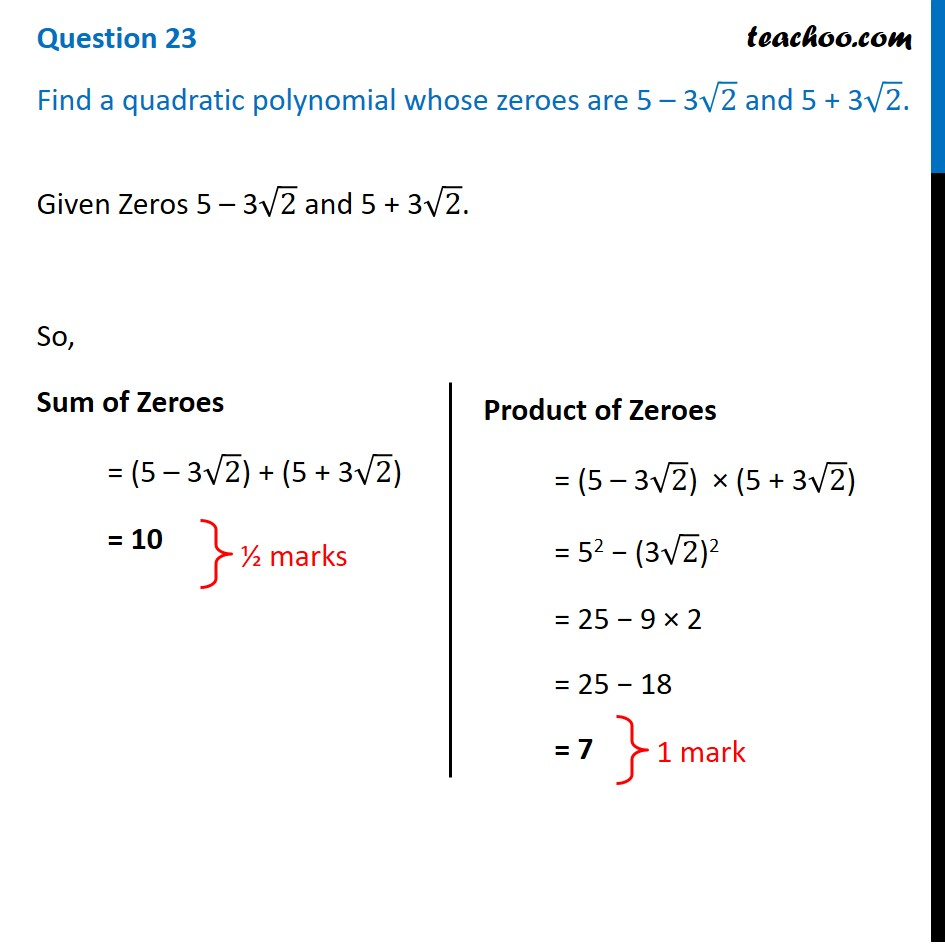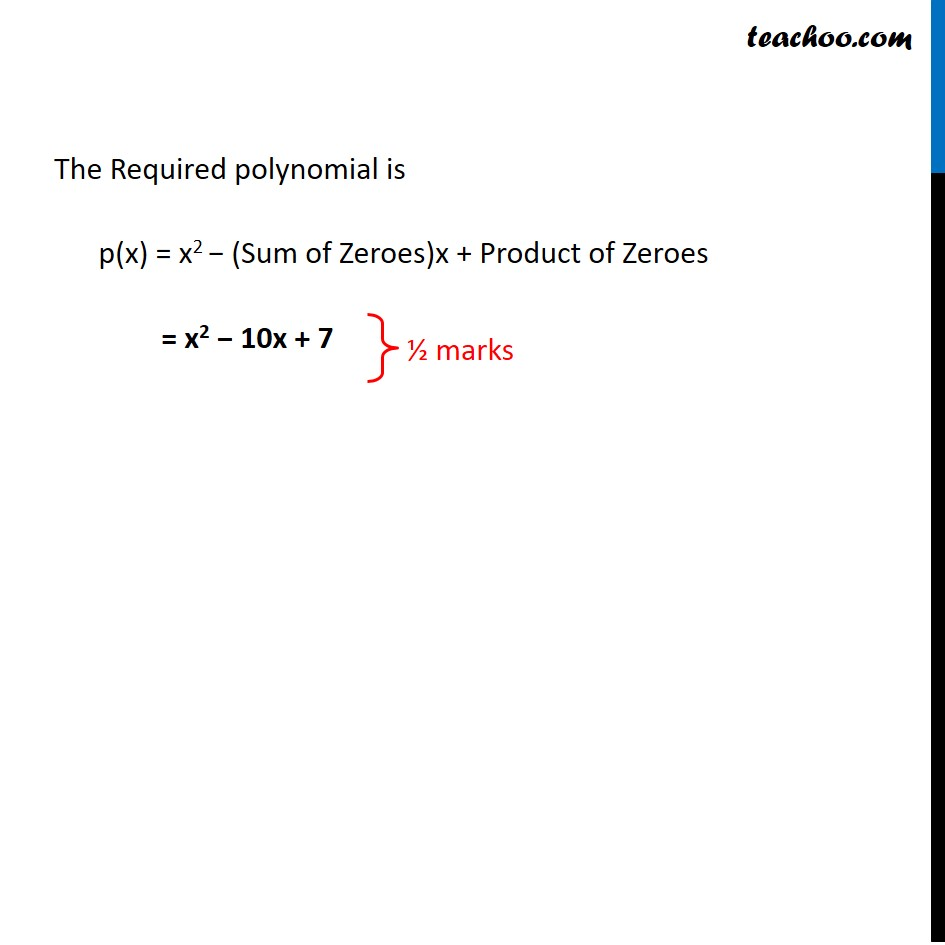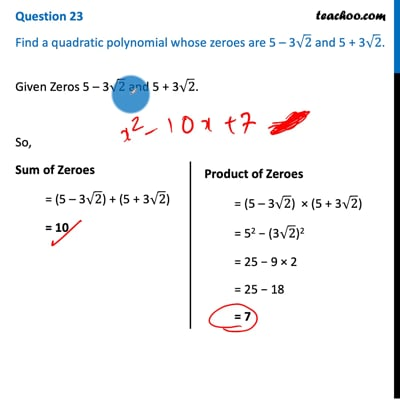CBSE Class 10 Sample Paper for 2021 Boards - Maths Standard

Class 10
Solutions of Sample Papers for Class 10 Boards

## Find a quadratic polynomial whose zeroes are 5 – 3√2 and 5 + 3√2.This video is only available for Teachoo black users

Introducing your new favourite teacher - Teachoo Black, at only ₹83 per month

### Transcript

Question 23 Find a quadratic polynomial whose zeroes are 5 – 3√2 and 5 + 3√2.Given Zeros 5 – 3√2 and 5 + 3√2. So, Sum of Zeroes = (5 – 3√2) + (5 + 3√2) = 10 Product of Zeroes = (5 – 3√2) × (5 + 3√2) = 52 − (3√2)2 = 25 − 9 × 2 = 25 − 18 = 7 The Required polynomial is p(x) = x2 − (Sum of Zeroes)x + Product of Zeroes = x2 − 10x + 7